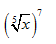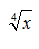#### MAT-HS.N-RN.01

 MAT-HS Targeted Standards(N) Concept: Number and Quantity(RN) Domain: The Real Number SystemCluster: Extend the properties of exponents to rational numbers MAT-HS.N-RN.01 Explain how the definition of the meaning of rational exponents follows from extending the properties of integer exponents to those values, allowing for a notation for radicals in terms of rational exponents. For example, we define 5 1/3 to be the cube root of 5 because we want (5 1/3)3 = 5(1/3)3 to hold, so (5 1/3)3 must equal 5.

## Student Learning Targets:

• I can
• I can

• I can
• I can

### Skills (Performance) Targets

• I can use exponential properties to explain how rational exponents follow from integer exponents.

• I can
• I can

## Alg II Convert Radicals to Rational Exponents Proficiency Scale

 Score Description Sample Activity 4.0 (advanced) In addition to Score 3.0, the student demonstrates in-depth inferences and applications regarding more complex material that go beyond end of instruction expectations. 3.5 In addition to Score 3.0 performance, the student demonstrates in-depth inferences and applications regarding the more complex content with partial success. 3.0 (proficient) The student can: rewrite exponents in radical notation and radicals in exponential notation. The student exhibits no major errors or omissions. Write x2/3 in radical notation. Writein exponential notation. 2.5 The student demonstrates no major errors or omissions regarding the simpler details and processes (Score 2.0 content) and partial knowledge of the more complex ideas and processes (Score 3.0 content). 2.0 (progressing) There are no major errors or omissions regarding the simpler details and processes as the student can:  Recognize and recall specific terminology, such as: radical and radical notation index rational exponent  However, the student exhibits major errors or omissions regarding the more complex ideas and processes. Write x1/3in radical notation. Rewritein exponential notation. 1.5 The student demonstrates partial knowledge of the simpler details and processes (Score 2.0 content) but exhibits major errors or omissions regarding the more complex ideas and procedures (Score 3.0 content). 1.0 (beginning) With help, the student demonstrates a partial understanding of some of the simpler details and processes (Score 2.0 content) and some of the more complex ideas and processes (Score 3.0 content). 0.5 With help, the student demonstrates a partial understanding of some of the simpler details and processes (Score 2.0 content) but not the more complex ideas and processes (Score 3.0 content). 0.0 Even with help, the student demonstrates no understanding or skill.

## Resources

 Web Vocab rational exponent